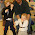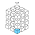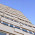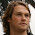Skip to main content

### Showing results from Cox Proportional Hazard Models in R with simPH

Update 2 February 2014: A new version of simPH (Version 1.0) will soon be available for download from CRAN. It allows you to plot using points, ribbons, and (new) lines. See the updated package description paper for examples. Note that the ribbons argument will no longer work as in the examples below. Please use type = 'ribbons' (or 'points' or 'lines').

Effectively showing estimates and uncertainty from Cox Proportional Hazard (PH) models, especially for interactive and non-linear effects, can be challenging with currently available software. So, researchers often just simply display a results table. These are pretty useless for Cox PH models. It is difficult to decipher a simple linear variable’s estimated effect and basically impossible to understand time interactions, interactions between variables, and nonlinear effects without the reader further calculating quantities of interest for a variety of fitted values.

So, I’ve been putting together the simPH R package to hopefully make it easier to show results from Cox PH models. The package uses plots of post-estimation simulations (the same idea behind the plotting facilities in the Zelig package) to show Cox PH estimates and the uncertainty surrounding them.

Here I want to give an overview of how to use simPH. First the general process, then a few examples. Have a look at this paper for details about the math behind the graphs.

### General Steps

There are three steps to use simPH:

1. Estimate a Cox PH model in the usual way with the coxph command in the survival package.

2. Simulate quantities of interest--hazard ratios, first differences, marginal effect, relative hazards, or hazard rates--with the appropriate simPH simulation command.

3. Plot the simulations with the simGG method.

### A Few Examples

Here are some basic examples that illustrate the process and key syntax. Before getting started you can install simPH in the normal R way from CRAN.

#### Linear effects

Let’s look at a simple example with a linear non-interactive effect. The data set I’m using is from Carpenter (2002). It is included with simPH. See the package documentation for details.

First, let’s estimate a Cox PH model where the event of interest is a drug receiving FDA approval.

# Load packages
library(survival)
library(simPH)

# Load Carpenter (2002) data
data("CarpenterFdaData")

# Estimate survival model
M1 <- coxph(Surv(acttime, censor) ~ prevgenx + lethal +
deathrt1 + acutediz + hosp01  + hhosleng +
mandiz01 + femdiz01 + peddiz01 + orphdum +
vandavg3 + wpnoavg3 + condavg3 + orderent +
stafcder, data = CarpenterFdaData)



Now say we want to examine the effect that the number of FDA staff reviewing a drug application has on it being accepted. This variable is called stafcder in the model we just estimated. To do this let’s use simPH to simulate hazard ratios. We will simulate hazard ratios for units $$j$$ and $$l$$, i.e. $$\frac{h_{j}(t)}{h_{l}(t)}$$ using simPH’s coxsimLinear command, because we estimate the effect of the number of staff as linear. In the following code we use the Xj argument to set the $$j$$ values. We could use Xl also, but as we don’t coxsimLinear assumes all $$x_{l}$$ are 0.

Notice that the argument spin = TRUE. This finds the shortest 95% probability interval of the simulations using the SPIn method. SPIn can be especially useful for showing simulated quantities of interest generated from Cox PH models, because then can often be crowded close to a lower boundary (0 in the case of hazard rates). We should be more interested in the area with the highest probability–most simulated values–rather than an arbitrary central interval. That being said, if spin = FALSE, then we will simply find the central 95% interval of the simulations.

# Simulate and plot Hazard Ratios for stafcder variable
Sim1 <- coxsimLinear(M1, b = "stafcder",
qi = "Hazard Ratio", ci = 0.95,
Xj = seq(1237, 1600, by = 2), spin = TRUE)
# Plot
simGG(Sim1)Notice in the plot that each simulation is plotted as an individual point. These are all of the simulations in the shortest 95% probability interval. Each point has a bit of transparency (they are 90% transparent by default). So the plot is visually weighted; the darker areas of the graph have a higher concentration of the simulations. This gives us a very clear picture of the simulation distribution, i.e. the estimated effect and our uncertainty about it.

If you don’t want to plot every point, you can simply use ribbons showing the constricted 95% and the middle 50% of this interval. To do this simply use the ribbons = TRUE argument.

simGG(Sim1, ribbons = TRUE, alpha = 0.5)Notice the alpha = 0.5 argument. This increased the transparency of the widest ribbon to 50%.

The syntax we’ve used here is very similar to what we use when we are working with nonlinear effects estimated with polynomials and splines. Post-estimation simulations can be run with the coxsimPoly and coxsimSpline commands. See the simPH documentation for more examples.

#### Interactions

The syntax for two-way interactions simulated with the coxsimInteract command is a little different. Using the same data, let’s look at how to show results for interactions. In the following model we are interacting two variables lethal and prevgenx. We can think of these variables as X1 and X2, respectively. For interactions it can be useful to examine the marginal effect. To find the marginal effect of a one unit increase in the lethal variable given various values of prevgenx let’s use the following code:

# Estimate the model
M2 <- coxph(Surv(acttime, censor) ~ lethal*prevgenx, data = CarpenterFdaData)

# Simulate Marginal Effect of lethal for multiple values of prevgenx
Sim2 <- coxsimInteract(M2, b1 = "lethal", b2 = "prevgenx",
qi = "Marginal Effect",
X2 = seq(2, 115, by = 2), nsim = 1000)

# Plot the results
simGG(Sim2, ribbons = TRUE, alpha = 0.5, xlab = "\nprevgenx",
ylab = "Marginal effect of lethal\n")The order of the X1 and X2 variables in the interactions matters. The marginal effect is always calculated for the X1 variable over a range of X2 values.

Notice also that we set the x and y axis labels with the xlab and ylab arguments.

#### Time-varying Effects

Finally, let’s look at how to use with the coxsimtvc command to show results from effects that we estimate to vary over time. Here we are going to use another data set that is also included with simPH. The event of interest in the following model is when deliberation is stopped on a European Union directive (the model is from Licht (2011)). We will create hazard ratios for the effect that the number of backlogged items (backlog) has on deliberation time. We will estimate the effect as a log-time interaction.

# Load Golub & Steunenberg (2007) data. The data is included with simPH.
data("GolubEUPData")

# Create natural log-time interactions
Golubtvc <- function(x){
tvc(data = GolubEUPData, b = x, tvar = "end", tfun = "log")
}
GolubEUPData$Lcoop <- Golubtvc("coop") GolubEUPData$Lqmv <- Golubtvc("qmv")
GolubEUPData$Lbacklog <- Golubtvc("backlog") GolubEUPData$Lcodec <- Golubtvc("codec")
GolubEUPData$Lqmvpostsea <- Golubtvc("qmvpostsea") GolubEUPData$Lthatcher <- Golubtvc("thatcher")

# Estimate model
M1 <- coxph(Surv(begin, end, event) ~ qmv + qmvpostsea + qmvpostteu +
coop + codec + eu9 + eu10 + eu12 + eu15 + thatcher +
agenda + backlog + Lqmv + Lqmvpostsea + Lcoop + Lcodec +
Lthatcher + Lbacklog,
data = GolubEUPData, ties = "efron")



Much of the first half of the code is dedicated to creating the log-time interactions with the tvc command.

Now we simply create the simulations for a range of values of backlog and plot them. Note in the following code that we tell coxsimtvc the name of both the base backlog variable and its log-time interaction term Lbacklog using the btvc argument. We also need to tell coxsimtvc that it is a log-time interaction with tfun = "log".

# Create simtvc object for relative hazard
Sim2 <- coxsimtvc(obj = M1, b = "backlog", btvc = "Lbacklog",
qi = "Relative Hazard", Xj = seq(40, 200, 40),
tfun = "log", from = 1200, to = 2000, by = 10,
nsim = 500)

# Create relative hazard plot
simGG(Sim2, xlab = "\nTime in Days", ribbons = TRUE,
leg.name = "Backlogged \n Items", alpha = 0.2)#### simGG Plots are ggplot2

Finally, because almost every plot created by simGG is a ggplot2 plot, you can use almost the full range of customisation options that that package allows. See the ggplot2 documentation for many examples.

### CommentsAdam Smith said…
This looks very handy! Thanks for the effort. Are you familiar with the mixed effects Cox models from the coxme package? I wonder how much work it would take to get your functions to work with the coxme objects? Probably wishful thinking...

Thanks again for these functions.Adam Smith said…
This comment has been removed by the author.Fr. said…
It's very fine code and it's much appreciated that you took the challenge to replicate existing work to illustrate the plotting routines.

Out of curiosity, how did that go? Did you manage to replicate what you wanted, and at the precision that you wanted?

I'm asking because I have sometimes obsessed about replicating exactly some (survey data) designs, just to see if it could be done :)Adam I'm really interested in adding frailties (and also capabilities for competing risks) . I did a brief attempt at adding frailties a few months ago, but decided to focus on the core functionality first.

I'm trying to remember what tripped me up, I think it was something to do with an indecision about how to incorporate frailties in the simulations. I'ld have to go back and take a look at it to be sure. Any thoughts on the issue would be much appreciated!

---

Fr. Replicating the time-varying covariate stuff was pretty straightforward as the original piece had well documented Stata code for creating a similar graph.

The example I give here for the linear and interactive effects are not directly from the original paper, but made clear examples. However, in the working paper I linked to I include a replication of a spline effect that was only shown in a results table, rather than graphed in the original paper. I did find some oddities, that I'm thinking of writing about in a different piece.Adam Smith said…
Christopher, I think the same problem plagues mixed effects models in general...namely, how best to incorporate the uncertainty from the random effects into predictions/confidence intervals, etc. I don't have the time to pour through the code right now, but I wonder if it's possible to simulate from, e.g., a coxme object and simply ignore the frailty, sort of like generating confidence intervals from a mixed effects model using only the fixed effects? Forgive me if this makes no sense in the PH framework; I'm afraid I'm only just getting into these frailty models... I'm happy to send some data/coxme output, if that's at all helpful.Adam: That makes a lot of sense. I did something similar for simPH's hazard rate capabilities. It just draws the simulations for the coefficients and then brings in the baseline hazard for calculating the quantities of interest.

I'm kind of unsatisfied with this, though. Surely our uncertainty about the baseline hazard is interesting. Same with the frailty terms. I just haven't come across a good way to do this yet. (If anyone has any suggestions, please pass them along).

If you have a simple coxme model/data to use for testing that would be great. I guess, just email it along (including the citation info you'ld want me to use of course).Mathijs de Vaan said…
This comment has been removed by the author.wonderful graphs. thanks.Ana Dilger said…
Hi,
First of all, this package is awesome! Thank you very much for doing it. Graphs look very nice and they really make the interpretation of cph models easier.
I just have a question. I like and I find extremely useful the inclusion of the rug plot in the x-axis which shows the distribution of the variable in the sample used to estimate the model. However, it is difficult to see it with the light blue colour. Is there any way I can change the colour?

Thanks a lot

### Slide: one function for lag/lead variables in data frames, including time-series cross-sectional data

I often want to quickly create a lag or lead variable in an R data frame. Sometimes I also want to create the lag or lead variable for different groups in a data frame, for example, if I want to lag GDP for each country in a data frame.I've found the various R methods for doing this hard to remember and usually need to look at old blogposts. Any time we find ourselves using the same series of codes over and over, it's probably time to put them into a function. So, I added a new command–slide–to the DataCombine R package (v0.1.5).Building on the shift function TszKin Julian posted on his blog, slide allows you to slide a variable up by any time unit to create a lead or down to create a lag. It returns the lag/lead variable to a new column in your data frame. It works with both data that has one observed unit and with time-series cross-sectional data.Note: your data needs to be in ascending time order with equally spaced time increments. For example 1995, 1996, 1997. ExamplesNot…

### A Link Between topicmodels LDA and LDAvis

Carson Sievert and Kenny Shirley have put together the really nice LDAvis R package. It provides a Shiny-based interactive interface for exploring the output from Latent Dirichlet Allocation topic models. If you've never used it, I highly recommend checking out their XKCD example (this paper also has some nice background).LDAvis doesn't fit topic models, it just visualises the output. As such it is agnostic about what package you use to fit your LDA topic model. They have a useful example of how to use output from the lda package.I wanted to use LDAvis with output from the topicmodels package. It works really nicely with texts preprocessed using the tm package. The trick is extracting the information LDAvis requires from the model and placing it into a specifically structured JSON formatted object.To make the conversion from topicmodels output to LDAvis JSON input easier, I created a linking function called topicmodels_json_ldavis. The full function is below. To use it follow …

### Dropbox & R Data

I'm always looking for ways to download data from the internet into R. Though I prefer to host and access plain-text data sets (CSV is my personal favourite) from GitHub (see my short paper on the topic) sometimes it's convenient to get data stored on Dropbox. There has been a change in the way Dropbox URLs work and I just added some functionality to the repmis R package. So I though that I'ld write a quick post on how to directly download data from Dropbox into R. The download method is different depending on whether or not your plain-text data is in a Dropbox Public folder or not.Dropbox Public FolderDropbox is trying to do away with its public folders. New users need to actively create a Public folder. Regardless, sometimes you may want to download data from one. It used to be that files in Public folders were accessible through non-secure (http) URLs. It's easy to download these into R, just use the read.table command, where the URL is the file name. Dropbox recent…